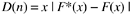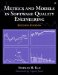# Modeling Process

To model software reliability, the following process or similar procedures should be used.

1. Examine the data. Study the nature of the data (fault counts versus times between failures), the unit of analysis (CPU hour , calendar day, week, month, etc.), the data tracking system, data reliability, and any relevant aspects of the data. Plot the data points against time in the form of a scatter diagram, analyze the data informally, and gain an insight into the nature of the process being modeled . For example, observe the trend, fluctuations, and any peculiar patterns and try to associate the data patterns with what was happening in the testing process. As another example, sometimes if the unit of time is too granular (e.g., calendar-time in hours of testing), the noise of the data may become too large relative to the underlying system pattern that we try to model. In that case, a larger time unit such as day or week may yield a better model.
2. Select a model or several models to fit the data based on an understanding of the test process, the data, and the assumptions of the models. The plot in step 1 can provide helpful information for model selection.
3. Estimate the parameters of the model. Different methods may be required depending on the nature of the data. The statistical techniques (e.g., the maximum likelihood method, the least-squares method, or some other method) and the software tools available for use should be considered .
4. Obtain the fitted model by substituting the estimates of the parameters into the chosen model. At this stage, you have a specified model for the data set.
5. Perform a goodness-of-fit test and assess the reasonableness of the model. If the model does not fit, a more reasonable model should be selected with regard to model assumptions and the nature of the data. For example, is the lack of fit due to a few data points that were affected by extraneous factors? Is the time unit too granular so that the noise of the data obscures the underlying trend?
6. Make reliability predictions based on the fitted model. Assess the reasonableness of the predictions based on other available informationactual performance of a similar product or of a previous release of the same product, subjective assessment by the development team, and so forth.

To illustrate the modeling process with actual data, the following sections give step-by-step details on the example shown in Figures 8.1 and 8.2. Table 8.1 shows the weekly defect rate data.

Step 1

The data were weekly defect data from the system test, the final phase of the development process. During the test process the software was under formal change con-trolany defects found are tracked by the electronic problem tracking reports (PTR) and any change to the code must be done through the PTR process, which is enforced by the development support system. Therefore, the data were reliable. The density plot and cumulative plot of the data are shown in Figures 8.1 and 8.2 (ignore temporarily the fitted curves).

Table 8.1. Weekly Defect Arrival Rates and Cumulative Rates

Week

Defects/KLOC Arrival

Defects/KLOC Cumulative

1

.353

.353

2

.436

.789

3

.415

1.204

4

.351

1.555

5

.380

1.935

6

.366

2.301

7

.308

2.609

8

.254

2.863

9

.192

3.055

10

.219

3.274

11

.202

3.476

12

.180

3.656

13

.182

3.838

14

.110

3.948

15

.155

4.103

16

.145

4.248

17

.221

4.469

18

.095

4.564

19

.140

4.704

20

.126

4.830

Step 2

The data indicated an overall decreasing trend (of course, with some noises), therefore the exponential model was chosen. For other products, we had used the delayed S and inflection S models. Also, the assumption of the S models, specifically the delayed reporting of failures due to problem determination and the mutual dependence of defects, seems to describe the development process correctly. However, from the trend of the data we did not observe an increase-then-decrease pattern, so we chose the exponential model. We did try the S models for goodness of fit, but they were not as good as the exponential model in this case.

Step 3

We used two methods for model estimation. In the first method, we used an SAS program similar to the one shown in Figure 7.5 in Chapter 7, which used a nonlinear regression approach based on the DUD algorithm (Ralston and Jennrich, 1978). The second method relies on the Software Error Tracking Tool (SETT) software developed by Falcetano and Caruso at IBM Kingston (Falcetano and Caruso, 1988). SETT implemented the exponential model and the two S models via the Marquardt non-linear least-squares algorithm. Results of the two methods are very close. From the DUD nonlinear regression methods, we obtained the following values for the two parameters K and l .

K = 6.597

l =0.0712

The asymptotic 95% confidence intervals for the two parameters are:

Lower

Upper

K

5.643

7.552

l

0.0553

0.0871

Step 4

By fitting the estimated parameters from step 3 into the exponential distribution, we obtained the following specified modelwhere t is the week number since the start of system test.

Step 5

We conducted the Kolmogorov-Smirnov goodness-of-fit test (Rohatgi, 1976) between the observed number of defects and the expected number of defects from the model in step 4. The Kolmogorov-Smirnov test is recommended for goodness-of-fit testing for software reliability models (Goel, 1985). The test statistic is as follows :where n is sample size , F *( x ) is the normalized observed cumulative distribution at each time point (normalized means the total is 1), and F ( x ) is the expected cumulative distribution at each time point, based on the model. In other words, the statistic compares the normalized cumulative distributions of the observed rates and the expected rates from the model at each point, then takes the absolute difference. If the maximum difference, D ( n ), is less than the established criteria, then the model fits the data adequately.

Table 8.2 shows the calculation of the test. Column (A) is the third column in Table 8.1. Column (B) is the cumulative defect rate from the model. The F *( x ) and F ( x ) columns are the normalization of columns (A) and (B), respectively. The maximum of the last column, F *( x ) - F ( x ), is .02329. The Kolmogorov-Smirnov test statistic for n = 20, and p value = .05 is .294 (Rohatgi, 1976, p. 661, Table 7). Because the D ( n ) value for our model is .02329, which is less than .294, the test indicates that the model is adequate.

Table 8.2. Weekly Defect Arrival Rates and Cumulative Rates

Week

Observed Defects/KLOC Model Defects/KLOC Cumulative (A)

Cumulative (B)

F *( x )

F ( x )

F *( x ) - F ( x )

1

.353

.437

.07314

.09050

.01736

2

.789

.845

.16339

.17479

.01140

3

1.204

1.224

.24936

.25338

.00392

4

1.555

1.577

.32207

.32638

.00438

5

1.935

1.906

.40076

.39446

.00630

6

2.301

2.213

.47647

.45786

.01861

7

2.609

2.498

.54020

.51691

.02329

8

2.863

2.764

.59281

.57190

.02091

9

3.055

3.011

.63259

.62311

.00948

10

3.274

3.242

.67793

.67080

.00713

11

3.476

3.456

.71984

.71522

.00462

12

3.656

3.656

.75706

.75658

.00048

13

3.838

3.842

.79470

.79510

.00040

14

3.948

4.016

.81737

.83098

.01361

15

4.103

4.177

.84944

.86438

.01494

16

4.248

4.327

.87938

.89550

.01612

17

4.469

4.467

.92515

.92448

.00067

18

4.564

4.598

.94482

.95146

.00664

19

4.704

4.719

.97391

.97659

.00268

20

4.830

4.832

1.00000

1.00000

.00000

D ( n ) = .02329

Step 6

We calculated the projected number of defects for the four years following completion of system test. The projection from this model was very close to the estimate from the Rayleigh model and to the actual field defect data.

At IBM Rochester we have been using the reliability modeling techniques for estimating the defect level of software products for some years. We found the Rayleigh, the exponential, and the two S-type models to have good applicability to AS/400's process and data. We also rely on cross-model reliability to assess the reasonableness of the estimates. Furthermore, historical data are used for model calibration and for adjustment of the estimates. Actual field defect data confirmed the predictive validity of this approach; the differences between actual numbers and estimates are small.Metrics and Models in Software Quality Engineering (2nd Edition)
ISBN: 0201729156
EAN: 2147483647
Year: 2001
Pages: 176